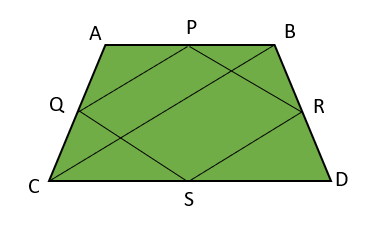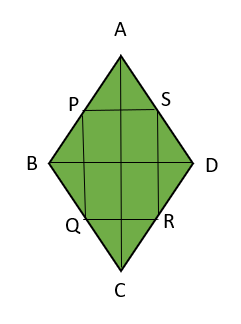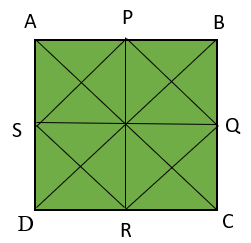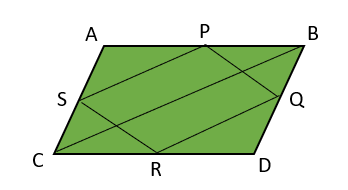Open in App
Not now

# What figure is created by joining the midpoints of any quadrilateral?

• Last Updated : 28 Mar, 2022

Geometry is derived from a Greek word that means ‘Earth Measurement’. It is a branch of mathematics and is concerned with the properties of space i.e., a visual study of shapes, position of figures, patterns, sizes, etc. Geometry is a subject of countless developments, so there exist many types. They are Euclidean Geometry, Non-Euclidean Geometry, Algebraic Geometry, Riemannian Geometry, and Symplectic Geometry.

A quadrilateral can be separated into two words Quad means four and lateral means side. So a quadrilateral is a closed figure with four sides. It had four vertices. The sides of a quadrilateral are equal/ unequal/ parallel/ irregular which leads to various geometric figures. Example: Square, Rectangle, Rhombus, Parallelogram, Trapezium, etc.

The midpoint of a side divides a side of any figure into two equal parts (length-wise). In a quadrilateral, there will be a midpoint for each side i.e., Four mid-points.

There are a few factors that determine the shape formed by joining the midpoints of a quadrilateral. Those factors are the kind of quadrilateral, diagonal properties, etc. These factors affect the shape formed by joining the midpoints in a given quadrilateral. Let’s look into the various scenarios to get a better understanding.

### What figure is created by joining the midpoints of any quadrilateral?

Solution:

Let’s consider a Quadrilateral ABCD, Find the shape of figure formed by joining the mid points.Here A, B, C, D are vertices of quadrilateral

P, Q, R, S are midpoints of sides AB, AC, BD and CD respectively.

As midpoint divides a side into equal parts, AP = PB and same will applied to all sides.

BC is the diagonal of the quadrilateral which form two triangles ABC and BCD.

Consider the Triangle BCD

CB is parallel to SR (CB || SR)

According to Mid-point theorem

SR = CB/2

So CB||SR and SR = CB/2 ⇢ (1)

Same as in Triangle ABC

QP||CB and QP = CB/2 ⇢ (2)

From (1) & (2)

SR||QP and SR = QP

As one pair of opposite sides are equal in length and parallel to each other, The resultant figure by joining the midpoints of a quadrilateral become a parallelogram.

### Sample Questions

Question 1: Consider the rhombus ABCD which is also a kind of Quadrilateral. Find the shape of the figure formed by joining the midpoints.

Solution:Let ABCD is a rhombus and P,Q,R,S are mid points of sides AB, BC, CD, DA respectively.

In Triangle ABD we have:

PS || BD & PS = BD/2            ….(1)

(According to midpoint theorem.)

In Triangle BDC we have

QR||BD & QR=BD/2               ….(2)

(According to mid point theorem.)

From equations (1) & (2) we get,

PS || QR

So PQRS is a parallelogram

As diagonals of rhombus bisect each other at 90° (Right angles)

Diagonals are perpendicular to each other

AC ⊥ BD

As PS || QR & PQ || SR & AC⊥ BD

PQ is also perpendicular to QR.

PQ ⊥ QR (∠PQR = 90°)

Hence PQRS is a Rectangle.

So, the figure formed by combining the midpoints of rhombus forms a rectangle.

Question 2: If the figure formed by joining the midpoint of a quadrilateral is square only if, do explain the condition.

Solution:Let ABCD is a rhombus and P, Q, R, S are mid points of sides AB, BC, CD, DA respectively.

Given PQRS is a Square.

Such that PQ = QR = RS = SP               ….(1)

Also diagonals in a square are of equal length i.e., PR = SQ

But PR = BC & SQ = AB

Hence AB = BC (as PR = SQ)

So all sides of quadrilateral are equal.

The given quadrilateral must be a square or rhombus.

RS||AC & RS = AC/2                            ….(2)

(According to midpoint theorem.)

In Triangle BDC we have

QR || BD & QR = BD/2                      …..(3)

(According to mid point theorem. 0

From equation (1),

RS = QR

So, AC/2 = BD/2

AC = BD

Thus, the length of diagonals of quadrilateral are same, So ABCD is a square with diagonals perpendicular to each other.

Question 3: What is the figure formed by joining the midpoints of a parallelogram.

Solution:The given figure is Parallelogram ABCD with midpoints P, Q, R, S for the sides AB, BD, CD, AC respectively.

In Triangle ABC we have,

PS || BC & PS = BC/2                 ….(1)

(According to midpoint theorem.)

In Triangle BDC we have:

QR || BC & QR = BC/2            ….(2)

(According to mid point theorem.)

From equation (1) & (2) we get,

PS || QR

So, PQRS is a parallelogram.

Question 4: What is the figure formed by joining the midpoints of a quadrilateral whose diagonals are of length equal?